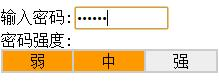# js密码强度校验，js数组常见操作及数组与字符串相互转化实例详解

JS截取字符串可使用 substring()或者slice()

``````<script type="text/javascript">
var obj="new1abcdefg".replace(/(.)(?=[^\$])/g,"\$1,").split(",");
//字符串转化为数组
var obj2 = "new2abcdefg".split("");  //字符串转化为数组
</script>
``````js数组的常见操作

``````<html>
<script language="javascript">
//CharMode函数
//测试某个字符是属于哪一类.
function CharMode(iN){
if (iN>=48 && iN <=57) //数字
return 1;
if (iN>=65 && iN <=90) //大写字母
return 2;
if (iN>=97 && iN <=122) //小写
return 4;
else
return 8; //特殊字符
}
//bitTotal函数
//计算出当前密码当中一共有多少种模式
function bitTotal(num){
modes=0;
for (i=0;i<4;i++){
if (num & 1) modes++;
num /= 2;
}
return modes;
}
//checkStrong函数
//返回密码的强度级别
function checkStrong(sPW){
if (sPW.length<=4)
return 0; //密码太短
Modes=0;
for (i=0;i<sPW.length;i++){
//测试每一个字符的类别并统计一共有多少种模式.
Modes|=CharMode(sPW.charCodeAt(i));
}
return bitTotal(Modes);
}
//pwStrength函数
//当用户放开键盘或密码输入框失去焦点时，根据不同的级别显示不同的颜色
function pwStrength(pwd){
O_color="#eeeeee";
L_color="#FF0000";
M_color="#FF9900";
H_color="#33CC00";
if (pwd==null||pwd==''){
Lcolor=Mcolor=Hcolor=O_color;
}else{
S_level=checkStrong(pwd);
switch(S_level) {
case 0:
Lcolor=Mcolor=Hcolor=O_color;
case 1:
Lcolor=L_color;
Mcolor=Hcolor=O_color;
break;
case 2:
Lcolor=Mcolor=M_color;
Hcolor=O_color;
break;
default:
Lcolor=Mcolor=Hcolor=H_color;
}
}
document.getElementById("strength_L").style.background=Lcolor;
document.getElementById("strength_M").style.background=Mcolor;
document.getElementById("strength_H").style.background=Hcolor;
}
</script>

<body>

<form>

<br>密码强度:
<table width="217" border="1" cellspacing="0" cellpadding="1" bordercolor="#cccccc" height="20">
<tr align="center" bgcolor="#eeeeee">
<td width="33%" id="strength_L">弱</td>
<td width="33%" id="strength_M">中</td>
<td width="33%" id="strength_H">强</td>
</tr>
</table>
</form>

</body>
</html>
``````

1、数组的创建

``````var arrayObj = new Array();　//创建一个数组
var arrayObj = new Array([size]);　//创建一个数组并指定长度，注意不是上限，是长度
var arrayObj = new Array([element0[, element1[, ...[, elementN]]]]);　创建一个数组并赋值
``````
``````var src="images/off_1.png";
//弹出值为：off
``````

#### 您可能感兴趣的文章:

• JS
密码强度验证(兼容IE,火狐,谷歌)
• js检验密码强度(低中高)附图
• Js密码强度实时验证代码
• javascript密码强度校验代码（两种方法）
• JavaScript
密码强度判断代码
• js检测用户输入密码强度
• js密码强度实时检测代码
• JavaScript判断密码强度(自写代码)
• javascript实现密码强度显示
• js实现密码强度检测【附示例】
• js正则表达式验证密码强度【推荐】
• js密码强度检测
• JavaScript动态检验密码强度的实现方法
• JS实现的四级密码强度检测功能示例

2、数组的元素的访问

``````var testGetArrValue=arrayObj; //获取数组的元素值
arrayObj= "这是新值"; //给数组元素赋予新的值
``````

3、数组元素的添加

``````arrayObj. push([item1 [item2 [. . . [itemN ]]]]);// 将一个或多个新元素添加到数组结尾，并返回数组新长度
arrayObj.unshift([item1 [item2 [. . . [itemN ]]]]);// 将一个或多个新元素添加到数组开始，数组中的元素自动后移，返回数组新长度
arrayObj.splice(insertPos,0,[item1[, item2[, . . . [,itemN]]]]);//将一个或多个新元素插入到数组的指定位置，插入位置的元素自动后移，返回""。
``````
``````var src="images/off_1.png";
//弹出值为：off
``````

4、数组元素的删除

``````arrayObj.pop(); //移除最后一个元素并返回该元素值
arrayObj.shift(); //移除最前一个元素并返回该元素值，数组中元素自动前移
arrayObj.splice(deletePos,deleteCount); //删除从指定位置deletePos开始的指定数量deleteCount的元素，数组形式返回所移除的元素
``````

5、数组的截取和合并

``````arrayObj.slice(start, [end]); //以数组的形式返回数组的一部分，注意不包括 end 对应的元素，如果省略 end 将复制
start 之后的所有元素
arrayObj.concat([item1[, item2[, . . . [,itemN]]]]); //将多个数组（也可以是字符串，或者是数组和字符串的混合）连接为一个数组，返回连接好的新的数组
``````
``````str="jpg|bmp|gif|ico|png";
arr=theString.split("|");
//arr是一个包含字符值"jpg"、"bmp"、"gif"、"ico"和"png"的数组
``````

6、数组的拷贝

``````arrayObj.slice(0); //返回数组的拷贝数组，注意是一个新的数组，不是指向
arrayObj.concat(); //返回数组的拷贝数组，注意是一个新的数组，不是指向
``````

7、数组元素的排序

``````arrayObj.reverse(); //反转元素（最前的排到最后、最后的排到最前），返回数组地址
arrayObj.sort(); //对数组元素排序，返回数组地址
``````
``````var delimitedString=myArray.join(delimiter);
var myList=new Array("jpg","bmp","gif","ico","png");
var portableList=myList.join("|");
//结果是jpg|bmp|gif|ico|png
``````

8、数组元素的字符串化

arrayObj.join(separator);
//返回字符串，这个字符串将数组的每一个元素值连接在一起，中间用 separator

toLocaleString 、toString 、valueOf：可以看作是join的特殊用法，不常用

``````var myString="JavaScript";
var w=myString.indexOf("v");w will be 2
var x=myString.indexOf("S");x will be 4
var y=myString.indexOf("Script");y will also be 4
var z=myString.indexOf("key");z will be -1
``````

1、length 属性

Length属性表示数组的长度，即其中元素的个数。因为数组的索引总是由0开始，所以一个数组的上下限分别是：0和length-1。和其他大多数语言不同的是，JavaScript数组的length属性是可变的，这一点需要特别注意。当length属性被设置得更大时，整个数组的状态事实上不会发生变化，仅仅是length属性变大；当length属性被设置得比原来小时，则原先数组中索引大于或等于length的元素的值全部被丢失。下面是演示改变length属性的例子：

``````var arr=[12,23,5,3,25,98,76,54,56,76];
//定义了一个包含10个数字的数组
arr.length=12; //增大数组的长度
arr.length=5; //将数组的长度减少到5，索引等于或超过5的元素被丢弃
arr.length=10; //将数组长度恢复为10
``````

``````var src="images/off_1.png";
//弹出值依次为：6,15
``````

JavaScript中可以使用一个未声明过的变量，同样，也可以使用一个未定义的数组元素（指索引超过或等于length的元素），这时，length属性的值将被设置为所使用元素索引的值加1。例如下面的代码：

``````var arr=[12,23,5,3,25,98,76,54,56,76];
arr=34;
``````

substr 方法

Array()形式创建的数组，其初始长度就是为0，正是对其中未定义元素的操作，才使数组的长度发生变化。

stringvar.substr(start [, length ])

2、prototype 属性

stringvar

objectName.prototype

objectName 参数是object对象的名称。

start